Author: Oscar Cronquist Article last updated on October 27, 2021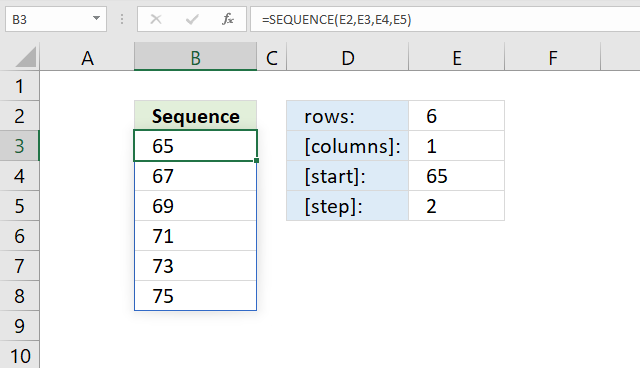The SEQUENCE function creates a list of sequential numbers to a cell range or array. It is located in the Math and trigonometry category and is only available to Excel 365 subscribers.

Formula in cell B3:

=SEQUENCE(E2,E3,E4,E5)

The formula demonstrated in the image above returns a sequence of six numbers from 65 to 75 with an incremental value of 2. 65, 67, 69, 71, 73, and 75.

## Excel Function Syntax

SEQUENCE(rows, [columns], [start], [step])

## Arguments

 Argument Text rows Required. Number of rows. [columns] Optional. Number of columns, 1 is the default value. [start] Optional. Start number, 1 is the default value. [step] Optional. A number to increment each value in the sequence.

## 1. What is a spilled array formula?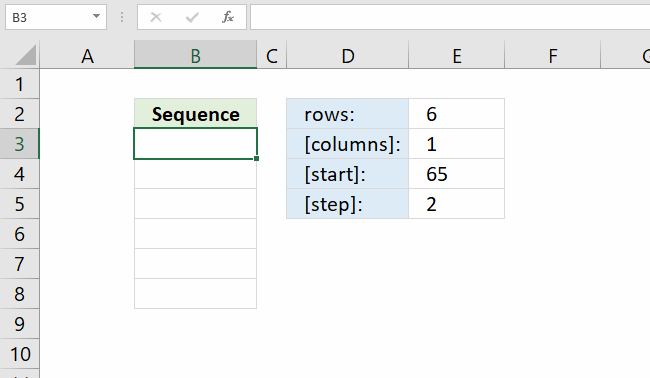Excel 365 automatically expands the output range based on the number of values in the array, without requiring the user to enter the formula as an array formula.

This new behavior of Excel is called spilled array formula and is something only dynamic array formulas can do. Dynamic array formulas are only available to Excel 365 subscribers.

## 2. Why does the SEQUENCE function return a #SPILL! error?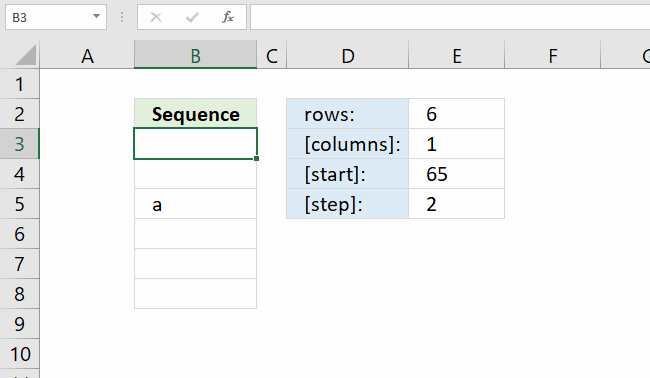#SPILL! error is returned by the SEQUENCE function if the required cell range is populated by any other value. You have two options:

• Remove value leaving the cell blank.
• Enter the dynamic formula in another cell that has empty adjacent cells.

## 3. Why does the SEQUENCE function return a #NAME! error?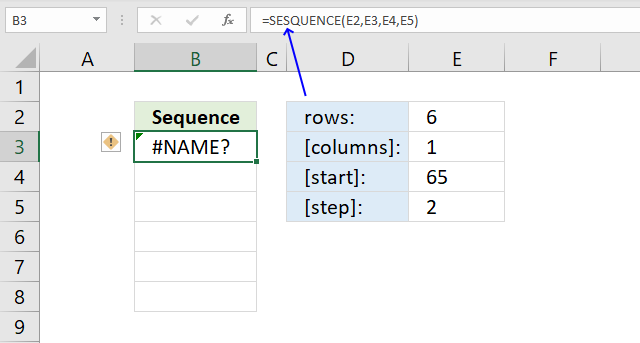If a cell returns #NAME! error you have either misspelled the function name or you are using an older incompatible Excel version.

The image above shows that I misspelled the SEQUENCE function in the formula bar, cell F3 displays #NAME! error.

Only Excel 365 subscription version supports the new dynamic array formula like the SEQUENCE function, older Excel versions like Excel 2019, 2016, 2013, 2010, 2007 and earlier versions do not support the SEQUENCE function.

Here is how to find out your Excel version: Get your Excel version

## 4. Create a dynamic calendar using the SEQUENCE function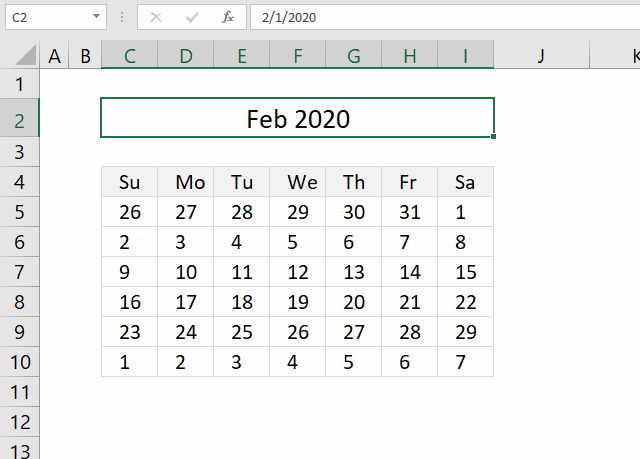The image above demonstrates a dynamic array formula in cell C5 that creates dates based on the date in cell C2. Cell E2 is formatted to only show the month and year.

Change the date in cell C2 and the dates in the calendar are instantly refreshed based on the new date value. The formula is a dynamic array formula and it returns an array of numbers, the array size is six rows and seven columns.

Formula in cell C5:

=SEQUENCE(6,7,C2-WEEKDAY(C2)+1)

### Explaining formula in cell C5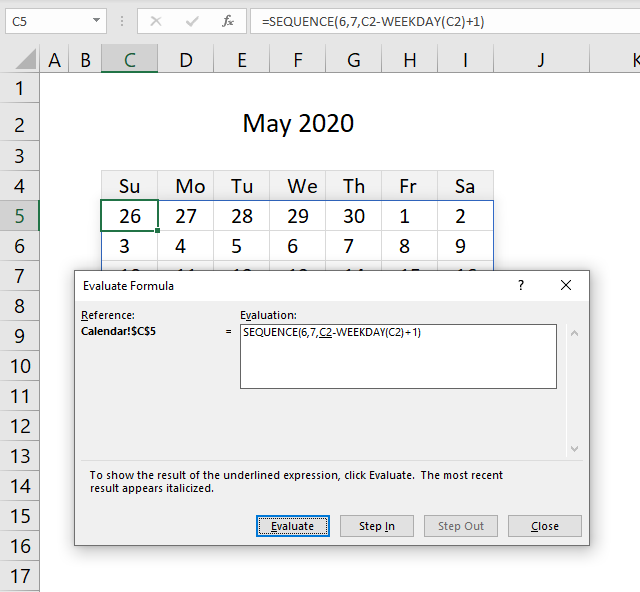#### Step 1 - Calculate weekday number

The WEEKDAY function converts an Excel date to a number 1 - 7 representing the weekday.

WEEKDAY(C2)

becomes

WEEKDAY(43952)

and returns 6. Friday is the sixth weekday in a week. The week begins with Sunday if you omit the second argument.

1 - Sunday
2- Monday
3- Tuesday
4-Wednesday
5-Thursday
6- Friday
7- Saturday

#### Step 2 - Calculate the first date in a week

This step calculates the date of the first day of the week. It is most often but not always a date in the last month.

C2-WEEKDAY(C2)+1

becomes

C2-6+1

becomes

43952-6+1

and returns 43947. This number represents a date in Excel. 1/1/1900 is 1, 43947 is 4/6/2020.

#### Step 3 - Create a sequence

SEQUENCE(6,7,C2-WEEKDAY(C2)+1)

becomes

SEQUENCE(6,7, 43947)

and returns

{43947, 43948, 43949, 43950, 43951, 43952, 43953;43954, 43955, 43956, 43957, 43958, 43959, 43960;43961, 43962, 43963, 43964, 43965, 43966, 43967;43968, 43969, 43970, 43971, 43972, 43973, 43974;43975, 43976, 43977, 43978, 43979, 43980, 43981;43982, 43983, 43984, 43985, 43986, 43987, 43988}.

## 5. How to create a repeating sequence?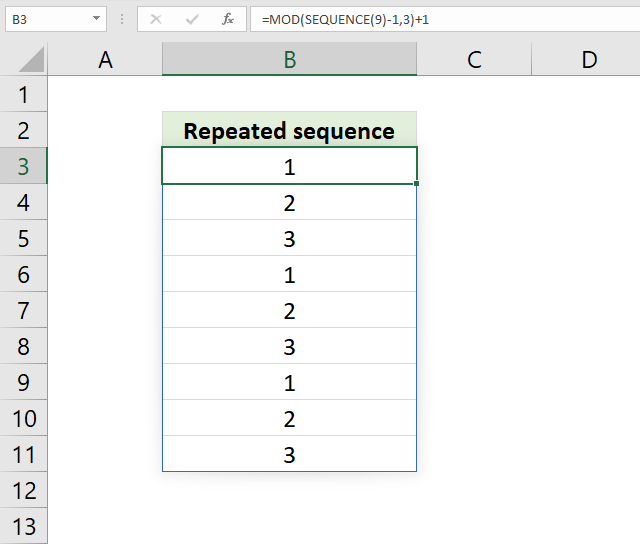The image above demonstrates a formula in cell B3 that creates a sequence from 1 to 3 repeated as far as necessary.

Formula in cell B3:

=MOD(SEQUENCE(9)-1,3)+1

### Explaining formula in cell C5

#### Step 1 - Create a sequence

SEQUENCE(9)-1

becomes

{1;2;3;4;5;6;7;8;9}-1

and returns {0; 1; 2; 3; 4; 5; 6; 7; 8}.

#### Step 2 - Calculate remainder

The MOD function returns the remainder after a number is divided by a divisor.

MOD(SEQUENCE(9)-1,3)+1

becomes

MOD({0; 1; 2; 3; 4; 5; 6; 7; 8} ,3)+1

becomes

{0; 1; 2; 0; 1; 2; 0; 1; 2}+1

and returns the following array

{1; 2; 3; 1; 2; 3; 1; 2; 3}.

## 6. How to create repeated numbers in a sequence?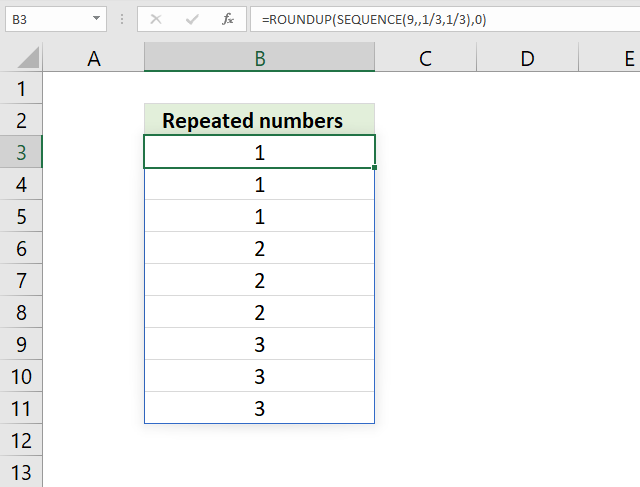The image above demonstrates a formula in cell B3 that creates a sequence of repeated numbers like 1, 1, 1, 2, 2, 2, 3, 3,...  as far as necessary.

Formula in cell B3:

=ROUNDUP(SEQUENCE(9, , 1/3, 1/3), 0)

### Explaining formula in cell C5

#### Step 1 - Create a sequence

SEQUENCE(rows, [columns], [start], [step])

SEQUENCE(9, , 1/3, 1/3)

returns

{0.333333333333333; 0.666666666666667; 1; 1.33333333333333; 1.66666666666667; 2; 2.33333333333333; 2.66666666666667; 3}

#### Step 2 - Round values up

The ROUNDUP function calculates a number rounded up based on the number of digits to which you want to round the number.

ROUNDUP(numbernum_digits)

ROUNDUP(SEQUENCE(9, , 1/3, 1/3), 0)

becomes

ROUNDUP({0.333333333333333; 0.666666666666667; 1; 1.33333333333333; 1.66666666666667; 2; 2.33333333333333; 2.66666666666667; 3}, 0)

and returns

{1; 1; 1; 2; 2; 2; 3; 3; 3}

## 7. How to create empty values in a sequence of numbers?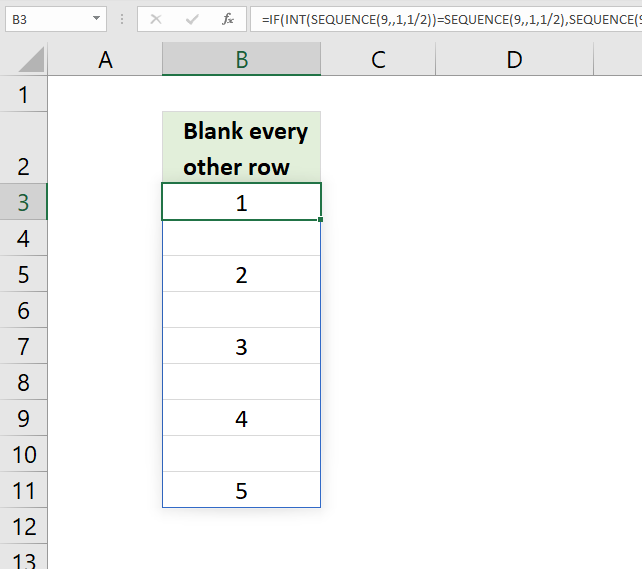The image above demonstrates a formula in cell B3 that creates a sequence from 1 to 5 with blanks in every other row.

Formula in cell B3:

=IF(INT(SEQUENCE(9, , 1, 1/2))=SEQUENCE(9, , 1, 1/2), SEQUENCE(9, , 1, 1/2), "")

### Explaining formula in cell C5

#### Step 1 - Create a sequence

SEQUENCE(9, , 1, 1/2)

returns {1; 1.5; 2; 2.5; 3; 3.5; 4; 4.5; 5}.

#### Step 2 - Create a logical expression

The INT function removes the decimal part from positive numbers.

INT(SEQUENCE(9, , 1, 1/2))=SEQUENCE(9, , 1, 1/2)

becomes

INT({1; 1.5; 2; 2.5; 3; 3.5; 4; 4.5; 5})={1; 1.5; 2; 2.5; 3; 3.5; 4; 4.5; 5}

becomes

{1; 1; 2; 2; 3; 3; 4; 4; 5}={1; 1.5; 2; 2.5; 3; 3.5; 4; 4.5; 5}

and returns

{TRUE; FALSE; TRUE; FALSE; TRUE; FALSE; TRUE; FALSE; TRUE}

#### Step 3 - Replace boolean values

IF(INT(SEQUENCE(9, , 1, 1/2))=SEQUENCE(9, , 1, 1/2), SEQUENCE(9, , 1, 1/2), "")

becomes

IF(INT({TRUE; FALSE; TRUE; FALSE; TRUE; FALSE; TRUE; FALSE; TRUE}, SEQUENCE(9, , 1, 1/2), "")

becomes

IF(INT({TRUE; FALSE; TRUE; FALSE; TRUE; FALSE; TRUE; FALSE; TRUE}, {1; 1.5; 2; 2.5; 3; 3.5; 4; 4.5; 5}, "")

and returns {1;"";2;"";3;"";4;"";5}.

## 8. How to format numbers in sequence with leading zeros?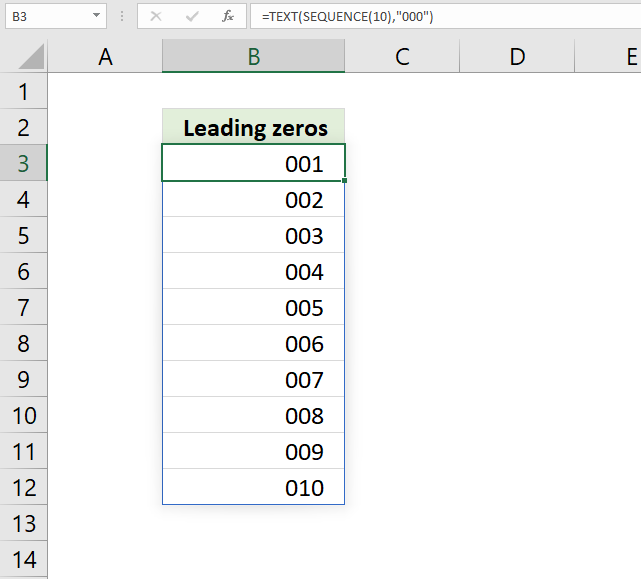The image above demonstrates a formula in cell B3 that creates a sequence from 1 to 1 with leading zeros.

Formula in cell B3:

=TEXT(SEQUENCE(10),"000")

### Explaining formula in cell C5

#### Step 1 - Create a sequence

SEQUENCE(10)

returns

{1; 2; 3; 4; 5; 6; 7; 8; 9; 10}

#### Step 2 - Format numbers

The TEXT function converts a value to text in a specific format. TEXT(value, format_text)

TEXT(SEQUENCE(10),"000")

becomes

TEXT({1; 2; 3; 4; 5; 6; 7; 8; 9; 10}, "000")

and returns

{"001"; "002"; "003"; "004"; "005"; "006"; "007"; "008"; "009"; "010"}.

## 9. How to reverse a list using the SEQUENCE function?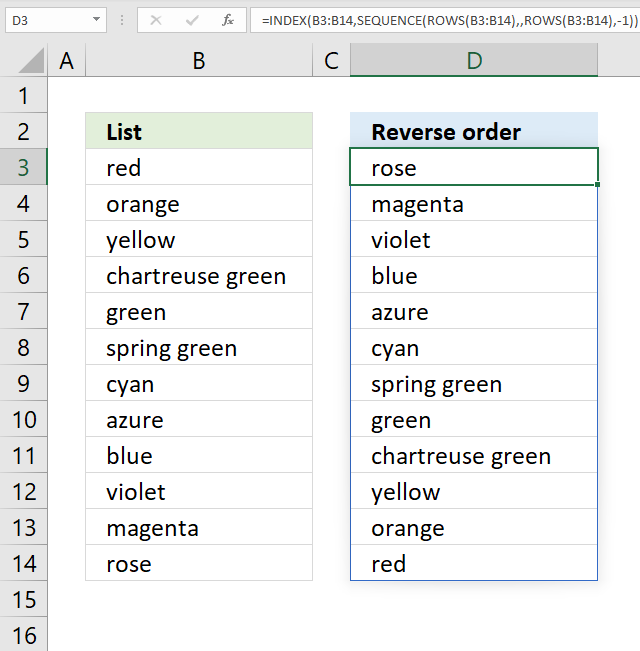The image above demonstrates a formula in cell D3 that flips data from cell range B3:B14.

Formula in cell B3:

=INDEX(B3:B14, SEQUENCE(ROWS(B3:B14), , ROWS(B3:B14), -1))

### Explaining formula in cell C5

The formula uses a cell reference to the old list in order to calculate a sequence that begins with a number representing the position of the last item in the list and then adds 1 to the next value until all values have been accounted for.

ROWS(B3:B14)

returns 12.

#### Step 2 - Create a sequence

The SEQUENCE function creates a sequence with start value 12 and increments by -1. SEQUENCE(rows, [columns], [start], [step])

SEQUENCE(ROWS(B3:B14), , ROWS(B3:B14), -1)

becomes

SEQUENCE(12, , 12, -1)

and returns {12; 11; 10; 9; 8; 7; 6; 5; 4; 3; 2; 1}.

#### Step 3 - Get values

The INDEX function returns values from a cell range based on a row and column arguments.

INDEX(B3:B14, SEQUENCE(ROWS(B3:B14), , ROWS(B3:B14), -1))

becomes

INDEX(B3:B14, {12; 11; 10; 9; 8; 7; 6; 5; 4; 3; 2; 1})

and returns

{"rose"; "magenta"; "violet"; "blue"; "azure"; "cyan"; "spring green"; "green"; "chartreuse green"; "yellow"; "orange"; "red"}.

## 10. Create sequential dates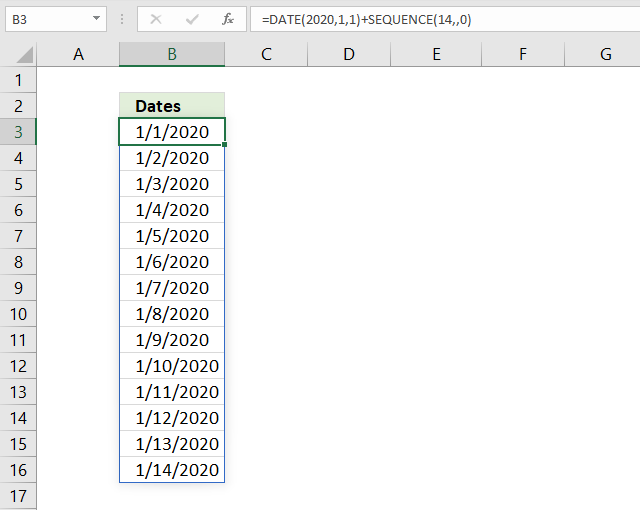The image above demonstrates a formula in cell D3 that creates a list of consecutive dates.

Formula in cell B3:

=DATE(2020,1,1)+SEQUENCE(14,,0)

### Explaining formula in cell C5

#### Step 1 - Create a sequence

The SEQUENCE function creates a sequence of 15 numbers from 0 (zero) to 14. SEQUENCE(rows, [columns], [start], [step])

SEQUENCE(14,,0)

returns

{0; 1; 2; 3; 4; 5; 6; 7; 8; 9; 10; 11; 12; 13}

#### Step 1 - Calculate rows in the cell reference

The DATE function returns an Excel date based on three arguments, year, month, and day. DATE(yearmonth, day)

DATE(2020,1,1)+SEQUENCE(14,,0)

becomes

DATE(2020,1,1)+{0; 1; 2; 3; 4; 5; 6; 7; 8; 9; 10; 11; 12; 13}

becomes

43831+{0; 1; 2; 3; 4; 5; 6; 7; 8; 9; 10; 11; 12; 13}

and returns

{43831; 43832; 43833; 43834; 43835; 43836; 43837; 43838; 43839; 43840; 43841; 43842; 43843; 43844}.

## 11. Create a sequence of months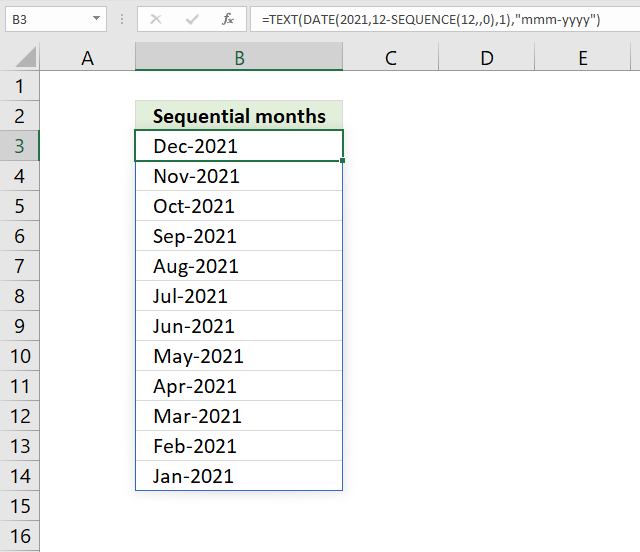The image above demonstrates a formula in cell D3 that flips data from cell range B3:B14.

Formula in cell B3:

=TEXT(DATE(2021,12-SEQUENCE(12,,0),1),"mmm-yyyy")

### Explaining formula in cell C5

#### Step 1 - Calculate rows in the cell reference

SEQUENCE(12, , 0) creates a sequence of 12 numbers that begins with 0 (zero) and ends with 11.

SEQUENCE(rows, [columns], [start], [step])

SEQUENCE(12, , 0)

returns

{0; 1; 2; 3; 4; 5; 6; 7; 8; 9; 10; 11}

#### Step 2 - Calculate rows in the cell reference

We are now going to use the array in the month argument, this creates an array of Excel dates beginning with 12-1-2021 and ending with 1-1-2021.

The DATE function creates Excel dates based on a given year, month, and days.

DATE(2021,12-SEQUENCE(12,,0),1)

becomes

DATE(2021,12-{0; 1; 2; 3; 4; 5; 6; 7; 8; 9; 10; 11}, 1)

and returns

{44531; 44501; 44470; 44440; 44409; 44378; 44348; 44317; 44287; 44256; 44228; 44197}.

#### Step 3 - Calculate rows in the cell reference

The TEXT function formats the Excel dates to mmm-yyyy.

TEXT(DATE(2021,12-SEQUENCE(12,,0),1),"mmm-yyyy")

becomes

TEXT({44531; 44501; 44470; 44440; 44409; 44378; 44348; 44317; 44287; 44256; 44228; 44197}, "mmm-yyyy")

and returns

{"Dec-2021"; "Nov-2021"; "Oct-2021"; "Sep-2021"; "Aug-2021"; "Jul-2021"; "Jun-2021"; "May-2021"; "Apr-2021"; "Mar-2021"; "Feb-2021"; "Jan-2021"}

## 12. Create a sequence of weeks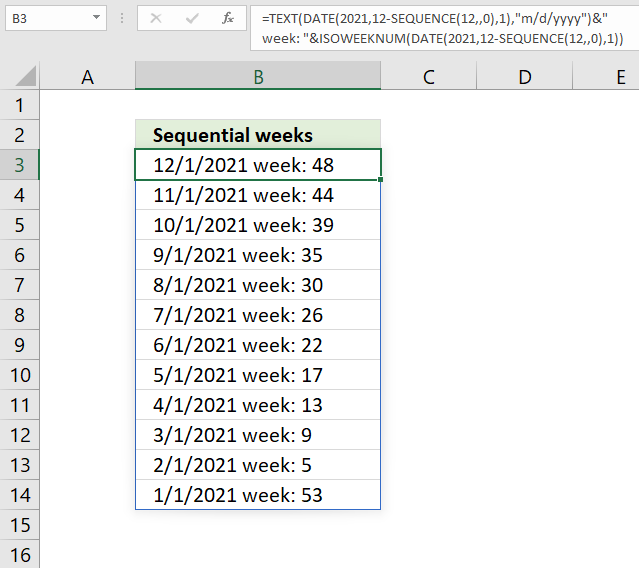The image above demonstrates a formula in cell D3 that flips data from cell range B3:B14.

Formula in cell B3:

=TEXT(DATE(2021, 12-SEQUENCE(12, , 0), 1), "m/d/yyyy")&" week: "&ISOWEEKNUM(DATE(2021, 12-SEQUENCE(12, , 0), 1))

### Explaining formula in cell C5

#### Step 1 - Create a sequence from 0 (zero) to 12

SEQUENCE(12, , 0)

returns

{0; 1; 2; 3; 4; 5; 6; 7; 8; 9; 10; 11}

#### Step 2 - Create a list of consecutive dates

The DATE function creates Excel dates based on a year, month, and day argument.

DATE(2021, 12-SEQUENCE(12, , 0), 1)

becomes

DATE(2021, 12-{0; 1; 2; 3; 4; 5; 6; 7; 8; 9; 10; 11}, 1)

becomes

DATE(2021,{12; 11; 10; 9; 8; 7; 6; 5; 4; 3; 2; 1},1)

and returns

{44531; 44501; 44470; 44440; 44409; 44378; 44348; 44317; 44287; 44256; 44228; 44197}

#### Step 3 - Change date formatting

The TEXT function formats the dates to m/d/yyyy.

TEXT(DATE(2021, 12-SEQUENCE(12, , 0), 1), "m/d/yyyy")

becomes

TEXT({44531; 44501; 44470; 44440; 44409; 44378; 44348; 44317; 44287; 44256; 44228; 44197}, "m/d/yyyy")

and returns

{"12/1/2021"; "11/1/2021"; "10/1/2021"; "9/1/2021"; "8/1/2021"; "7/1/2021"; "6/1/2021"; "5/1/2021"; "4/1/2021"; "3/1/2021"; "2/1/2021"; "1/1/2021"}

#### Step 4 - Calculate week number based on date

The ISOWEEKNUM function creates a week number of an Excel date.

ISOWEEKNUM(DATE(2021, 12-SEQUENCE(12, , 0), 1))

becomes

ISOWEEKNUM({44531; 44501; 44470; 44440; 44409; 44378; 44348; 44317; 44287; 44256; 44228; 44197})

and returns

{48; 44; 39; 35; 30; 26; 22; 17; 13; 9; 5; 53}

#### Step 5 - Concatenate date, text, and week values

The ampersand character & allows you to concatenate text string, numbers, arrays and so on.

TEXT(DATE(2021, 12-SEQUENCE(12, , 0), 1), "m/d/yyyy")&" week: "&ISOWEEKNUM(DATE(2021, 12-SEQUENCE(12, , 0), 1))

becomes

TEXT(DATE(2021, 12-SEQUENCE(12, , 0), 1), "m/d/yyyy")&" week: "&{48; 44; 39; 35; 30; 26; 22; 17; 13; 9; 5; 53}

becomes

{"12/1/2021"; "11/1/2021"; "10/1/2021"; "9/1/2021"; "8/1/2021"; "7/1/2021"; "6/1/2021"; "5/1/2021"; "4/1/2021"; "3/1/2021"; "2/1/2021"; "1/1/2021"}&" week: "&{48; 44; 39; 35; 30; 26; 22; 17; 13; 9; 5; 53}

and returns

{"12/1/2021 week: 48"; "11/1/2021 week: 44"; "10/1/2021 week: 39"; "9/1/2021 week: 35"; "8/1/2021 week: 30"; "7/1/2021 week: 26"; "6/1/2021 week: 22"; "5/1/2021 week: 17"; "4/1/2021 week: 13"; "3/1/2021 week: 9"; "2/1/2021 week: 5"; "1/1/2021 week: 53"}

## 13. Create a sequence of quarters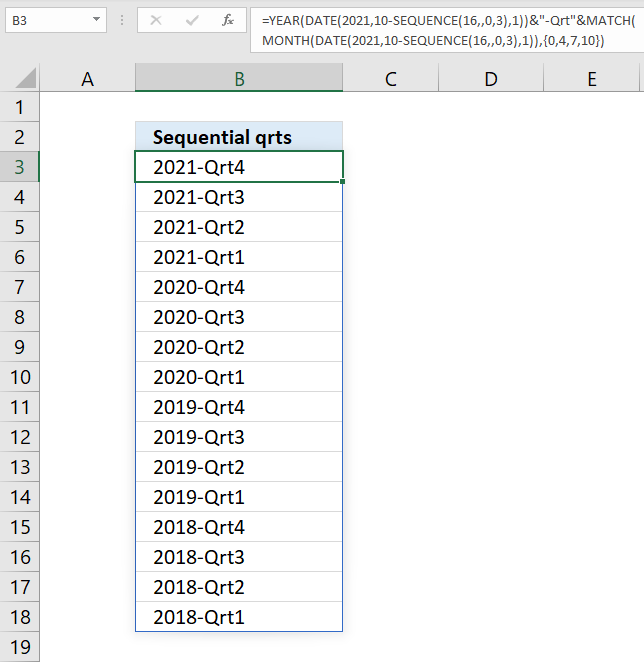Formula in cell B3:

=YEAR(DATE(2021,10-SEQUENCE(16,,0,3),1))&"-Qrt"&MATCH(MONTH(DATE(2021,10-SEQUENCE(16,,0,3),1)),{0,4,7,10})

### Explaining formula in cell C5

#### Step 1 - Create a sequence

SEQUENCE(16,,0,3)

returns

{0; 3; 6; 9; 12; 15; 18; 21; 24; 27; 30; 33; 36; 39; 42; 45}

#### Step 2 - Create a sequence of dates

The DATE function creates Excel dates using a year, month and day argument.

DATE(2021,10-SEQUENCE(16,,0,3),1)

becomes

DATE(2021,10-{0; 3; 6; 9; 12; 15; 18; 21; 24; 27; 30; 33; 36; 39; 42; 45},1)

and returns

{44470; 44378; 44287; 44197; 44105; 44013; 43922; 43831; 43739; 43647; 43556; 43466; 43374; 43282; 43191; 43101}

#### Step 3 - Convert dates to years

The YEAR function returns the year of an Excel date.

YEAR(DATE(2021,10-SEQUENCE(16,,0,3),1))

becomes

YEAR({44470; 44378; 44287; 44197; 44105; 44013; 43922; 43831; 43739; 43647; 43556; 43466; 43374; 43282; 43191; 43101})

and returns

{2021; 2021; 2021; 2021; 2020; 2020; 2020; 2020; 2019; 2019; 2019; 2019; 2018; 2018; 2018; 2018}

#### Step 4 - Convert dates to months

The MONTH function returns a number representing the relative position. 1 - January, ... , 12 - December.

MONTH(DATE(2021,10-SEQUENCE(16,,0,3),1))

becomes

MONTH({44470; 44378; 44287; 44197; 44105; 44013; 43922; 43831; 43739; 43647; 43556; 43466; 43374; 43282; 43191; 43101})

and returns

{10; 7; 4; 1; 10; 7; 4; 1; 10; 7; 4; 1; 10; 7; 4; 1}.

#### Step 5 - Convert months to quarters

The MATCH function returns the relative position of a given value in an array or cell range.

MATCH(MONTH(DATE(2021,10-SEQUENCE(16,,0,3),1)),{0,4,7,10})

becomes

MATCH({10; 7; 4; 1; 10; 7; 4; 1; 10; 7; 4; 1; 10; 7; 4; 1},{0,4,7,10})

and returns

{4; 3; 2; 1; 4; 3; 2; 1; 4; 3; 2; 1; 4; 3; 2; 1}

#### Step 6 - Concatenate year, text string and quarter number

The ampersand character & allows you to concatenate text string, numbers, arrays and so on.

YEAR(DATE(2021,10-SEQUENCE(16,,0,3),1))&"-Qrt"&MATCH(MONTH(DATE(2021,10-SEQUENCE(16,,0,3),1)),{0,4,7,10})

becomes

{2021; 2021; 2021; 2021; 2020; 2020; 2020; 2020; 2019; 2019; 2019; 2019; 2018; 2018; 2018; 2018}&"-Qrt"&MATCH(MONTH(DATE(2021,10-SEQUENCE(16,,0,3),1)),{0,4,7,10})

becomes

{2021; 2021; 2021; 2021; 2020; 2020; 2020; 2020; 2019; 2019; 2019; 2019; 2018; 2018; 2018; 2018}&"-Qrt"&{4; 3; 2; 1; 4; 3; 2; 1; 4; 3; 2; 1; 4; 3; 2; 1}

and returns

{"2021-Qrt4";"2021-Qrt3";"2021-Qrt2";"2021-Qrt1";"2020-Qrt4";"2020-Qrt3";"2020-Qrt2";"2020-Qrt1";"2019-Qrt4";"2019-Qrt3";"2019-Qrt2";"2019-Qrt1";"2018-Qrt4";"2018-Qrt3";"2018-Qrt2";"2018-Qrt1"}

## 14. Create a sequence of letters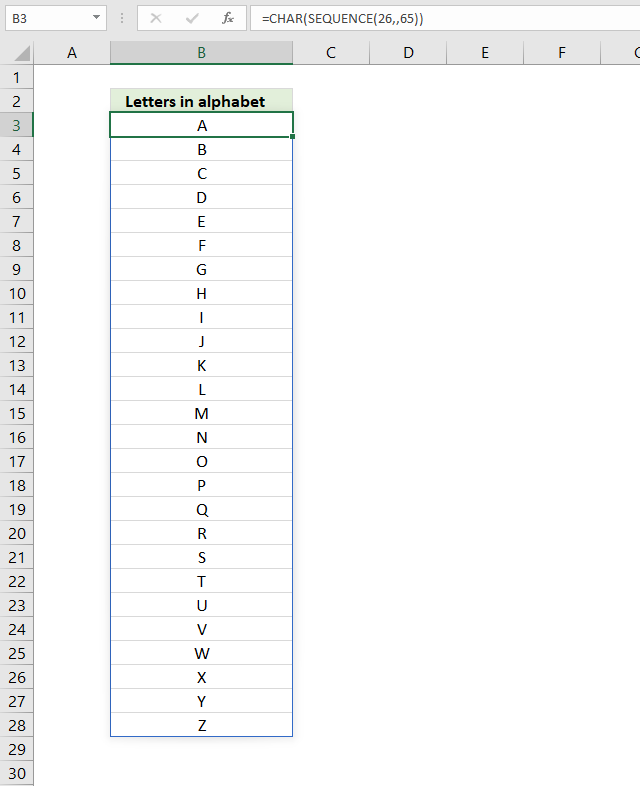The image above demonstrates a formula in cell B3 that creates letters from A to Z.

Formula in cell B3:

=CHAR(SEQUENCE(26,,65))

### Explaining formula in cell C5

#### Step 1 - Create a sequence of 26 numbers starting with 65

SEQUENCE(26,,65)

returns

{65; 66; 67; 68; 69; 70; 71; 72; 73; 74; 75; 76; 77; 78; 79; 80; 81; 82; 83; 84; 85; 86; 87; 88; 89; 90}

#### Step 2 - Convert numbers to characters

The CHAR function converts a number to the corresponding character which is determined by your computer's character set.

CHAR(SEQUENCE(26,,65))

becomes

CHAR({65; 66; 67; 68; 69; 70; 71; 72; 73; 74; 75; 76; 77; 78; 79; 80; 81; 82; 83; 84; 85; 86; 87; 88; 89; 90})

and returns

{"A"; "B"; "C"; "D"; "E"; "F"; "G"; "H"; "I"; "J"; "K"; "L"; "M"; "N"; "O"; "P"; "Q"; "R"; "S"; "T"; "U"; "V"; "W"; "X"; "Y"; "Z"}

## 15. Create a sequence of cells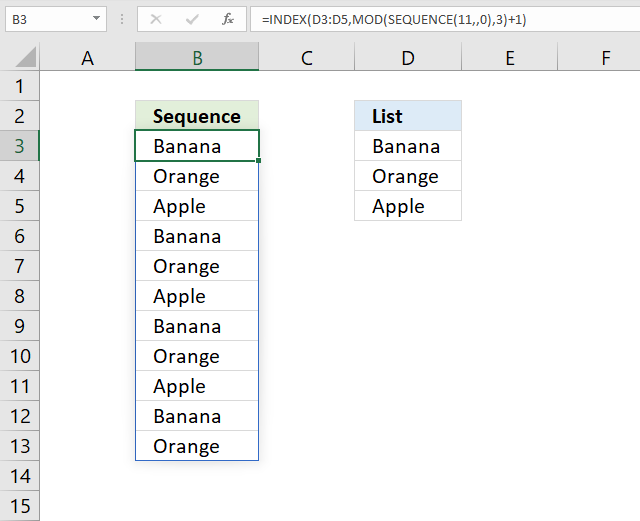The image above shows a formula in cell B3 that returns values from given a list and repeats those values four times.

Formula in cell B3:

=INDEX(D3:D5,MOD(SEQUENCE(11,,0),3)+1)

### Explaining formula in cell C5

#### Step 1 - Create a sequence from 0 (zero) to 11

To create a sequence from 0 (zero) to 11 we need to specify the first and third argument.

SEQUENCE(rows, [columns], [start], [step])

SEQUENCE(11, , 0)

returns {0; 1; 2; 3; 4; 5; 6; 7; 8; 9; 10}.

#### Step 2 - Create a repeating list of numbers

The MOD function returns the remainder after a number is divided by a divisor. MOD(number, divisor)

MOD(SEQUENCE(11,,0),3)

becomes

MOD({0; 1; 2; 3; 4; 5; 6; 7; 8; 9; 10}, 3)

and returns

{0; 1; 2; 0; 1; 2; 0; 1; 2; 0; 1}

#### Step 3 - Get values from cell range

The INDEX function returns values from a cell range based on a row and column arguments.

INDEX(D3:D5,MOD(SEQUENCE(11,,0),3)+1)

becomes

INDEX(D3:D5, {0; 1; 2; 0; 1; 2; 0; 1; 2; 0; 1}+1)

becomes

INDEX(D3:D5, {1; 2; 3; 1; 2; 3; 1; 2; 3; 1; 2})

and returns

{"Banana"; "Orange"; "Apple"; "Banana"; "Orange"; "Apple"; "Banana"; "Orange"; "Apple"; "Banana"; "Orange"}.

## 16. SEQUENCE function alternative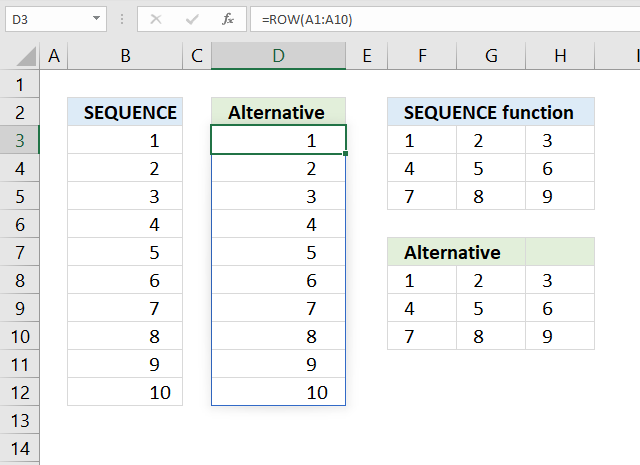The image above shows the SEQUENCE function in cell B3 and F3, cell D3 and F8 demonstrates alternative ways to create arrays if you can't use the SEQUENCE function.

The alternative formula in cell D3 creates an array of numbers from 1 to 10 in one column. The other alternative formula in cell F8 creates an array of numbers from 1 to 9 in three columns and three rows.

Formula in cell B3:

=SEQUENCE(10)

Alternative formula in cell D3:

=ROW(A1:A10)

Formula in cell F3:

=SEQUENCE(3,3)

Alternative formula in cell F8:

=COLUMN(A1:C1)+(ROW(A1:A3)-1)*3

### Get the Excel fileSEQUENCE-function.xlsx